# Model for Next Page propensity using Markov Chain model.# Model for Next Page propensity using Markov Chain model.

Andrey Markov first introduced Markov chains in the year 1906. He explained Markov chains as: A stochastic process containing random variables, transitioning from one state to another depending on certain assumptions and definite probabilistic rules. These random variables transition from one to state to the other, based on an important mathematical property called Markov Property.

## INTRODUCTION TO MARKOV CHAIN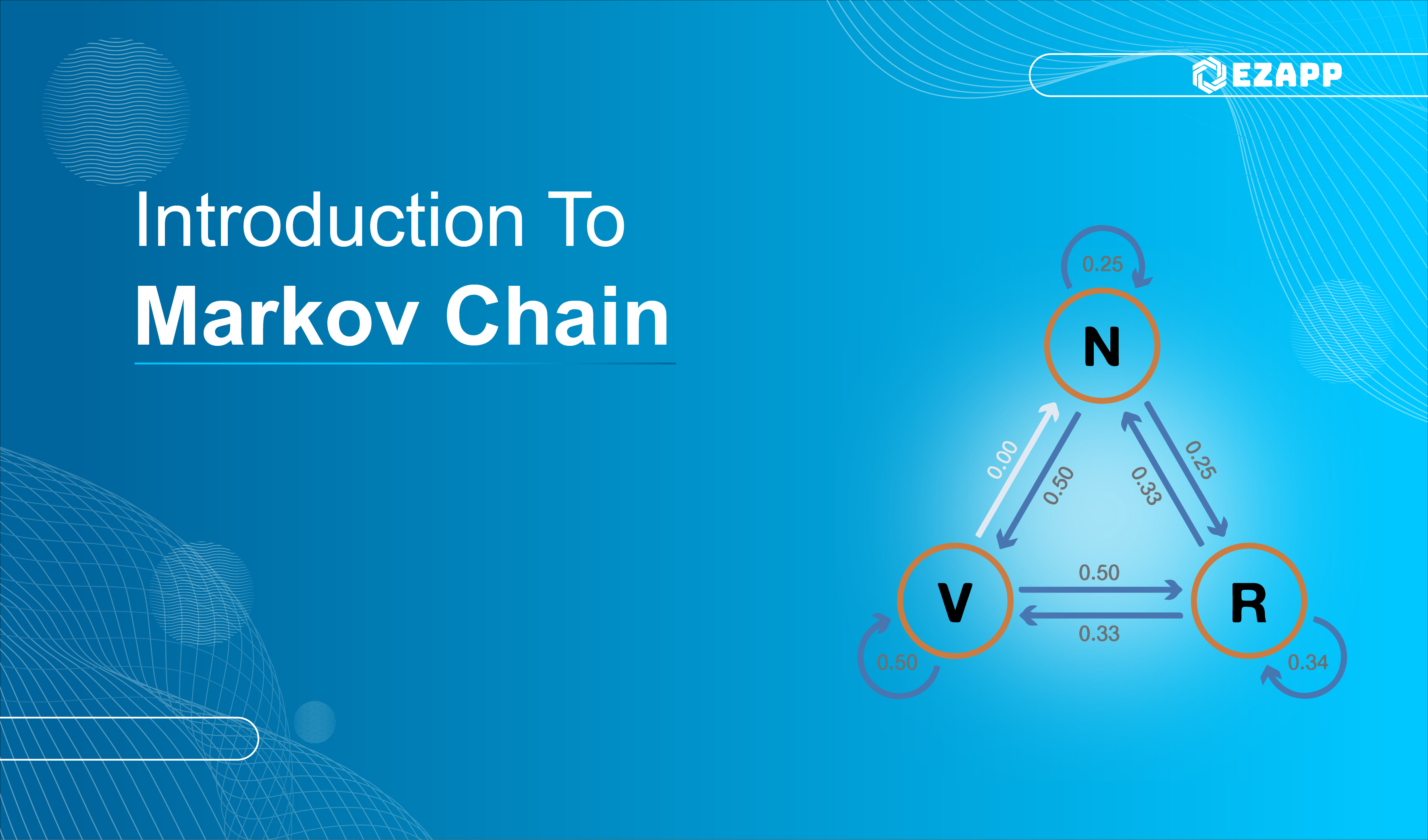Markov chain is a stochastic model portraying a succession of possible events in which the probability of every event relies just upon the state achieved in the previous event. A countably endless arrangement, in which the chain moves state at discrete time steps, gives a discrete-time Markov chain. A continuous-time process  is known as a continuous-time Markov chain. It is named after the Russian mathematician Andrey Markov.

Markov chains have many applications as statistical models of real-world processes, such as studying cruise control systems in motor vehicles, queues or lines of customers arriving at an airport, currency exchange rates and animal population dynamics.

Markov measures are the reason for general stochastic simulation methods known as Markov chain Monte Carlo, which are utilized for simulating sampling from complex likelihood disseminations, and have found application in Bayesian statistics, thermodynamics, statistical mechanics, physics, chemistry, economics, finance, signal processing, information theory and speech processing.

The modifier Markovian is utilized to depict something that is identified with a Markov process.

### What Is The Markov Property?

Discrete Time Markov Property states that the calculated probability of a random process transitioning to the next possible state is only dependent on the current state and time and it is independent of the series of states that preceded it.

The fact that the next possible action/ state of a random process does not depend on the sequence of prior states, renders Markov chains as a memory-less process that solely depends on the current state/action of a variable.

Let’s derive this mathematically:

Let the random process be, {Xm, m=0,1,2,⋯}.

This process is a Markov chain only if,

Markov Chain Formula - Introduction To Markov Chains - EzappSolution

Markov Chain – Introduction To Markov Chains – EzappSolution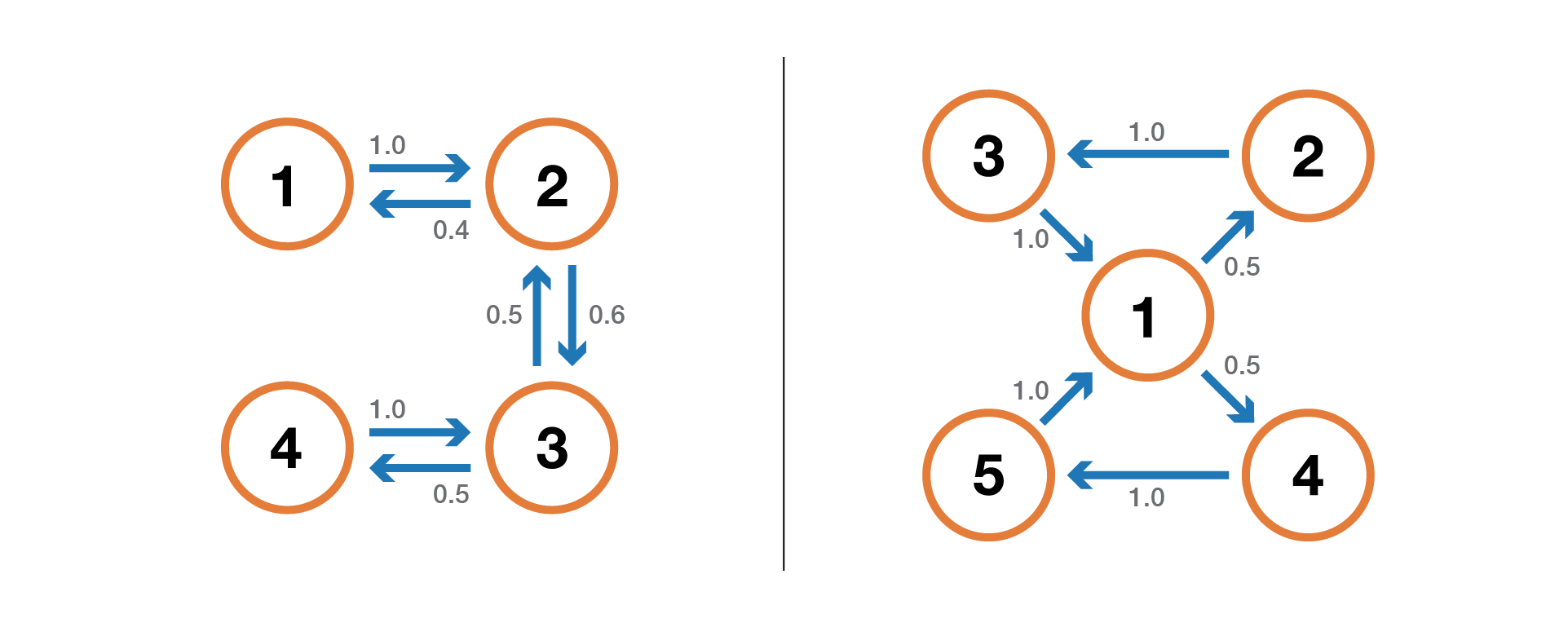for all m, j, i, i0, i1, ⋯ im−1

For a finite number of states, S={0, 1, 2, ⋯, r}, this is called a finite Markov chain.

P(Xm+1 = j|Xm = i) here represents the transition probabilities to transition from one state to the other. Here, we’re assuming that the transition probabilities are independent of time.

Which means that P(Xm+1 = j|Xm = i) does not depend on the value of ‘m’. Therefore, we can summarise,

Markov Chain - Introduction To Markov Chains - EzappSolution

Markov Chain Formula – Introduction To Markov Chains – EzappSolution

So this equation represents the Markov chain.

## HOW DOES MARKOV CHAIN WORK?

A Markov chain is represented using a probabilistic automaton (It only sounds complicated!). The changes of state of the system are called transitions. The probabilities associated with various state changes are called transition probabilities. A probabilistic automaton includes the probability of a given transition into the transition function, turning it into a transition matrix.

You can think of it as a sequence of directed graphs, where the edges of graph n are labeled by the probabilities of going from one state at time n to the other states at time n+1, Pr(Xn+1 = x | Xn = xn). You can read this as, probability of going to state Xn+1 given value of state Xn. The same information is represented by the transition matrix from time n to time n+1. Every state in the state space is included once as a row and again as a column, and each cell in the matrix tells you the probability of transitioning from its row's state to its column's state.

If the Markov chain has N possible states, the matrix will be an N x N matrix, such that entry (I, J) is the probability of transitioning from state I to state J. Additionally, the transition matrix must be a stochastic matrix, a matrix whose entries in each row must add up to exactly 1. Why? Since each row represents its own probability distribution.

So, the model is characterized by a state space, a transition matrix describing the probabilities of particular transitions, and an initial state across the state space, given in the initial distribution.

• Reducibility:  A Markov chain is said to be irreducible if it is possible to get to any state from any state. In other words, a Markov chain is irreducible if there exists a chain of steps between any two states that has positive probability.

• Periodicity:  A state in a Markov chain is periodic if the chain can return to the state only at multiples of some integer larger than 1. Thus, starting in state 'i', the chain can return to 'i' only at multiples of the period 'k', and k is the largest such integer. State 'i' is aperiodic if k = 1 and periodic if k > 1.

• Transience and Recurrence: A state 'i' is said to be transient if, given that we start in state 'i', there is a non-zero probability that we will never return to 'i'. State i is recurrent (or persistent) if it is not transient. A recurrent state is known as positive recurrent if it is expected to return within a finite number of steps and null recurrent otherwise.

• Ergodicity: A state 'i' is said to be ergodic if it is aperiodic and positive recurrent. If all states in an irreducible Markov chain are ergodic, then the chain is said to be ergodic.

• Absorbing State: A state i is called absorbing if it is impossible to leave this state. Therefore, the state 'i' is absorbing if pii = 1 and pij = 0 for i ≠ j. If every state can reach an absorbing state, then the Markov chain is an absorbing Markov chain.

## Solution

Once you have identified the problem. It is time to put the solution using python code.Now that we are familiar with the Markov Chain algorithm, let’s look at how we can fit Markov Chain models in Python

The Numpy Python machine learning library provides an implementation of Markov Chain for machine learning.

### IMPORT THE REQUIRED LIBRARY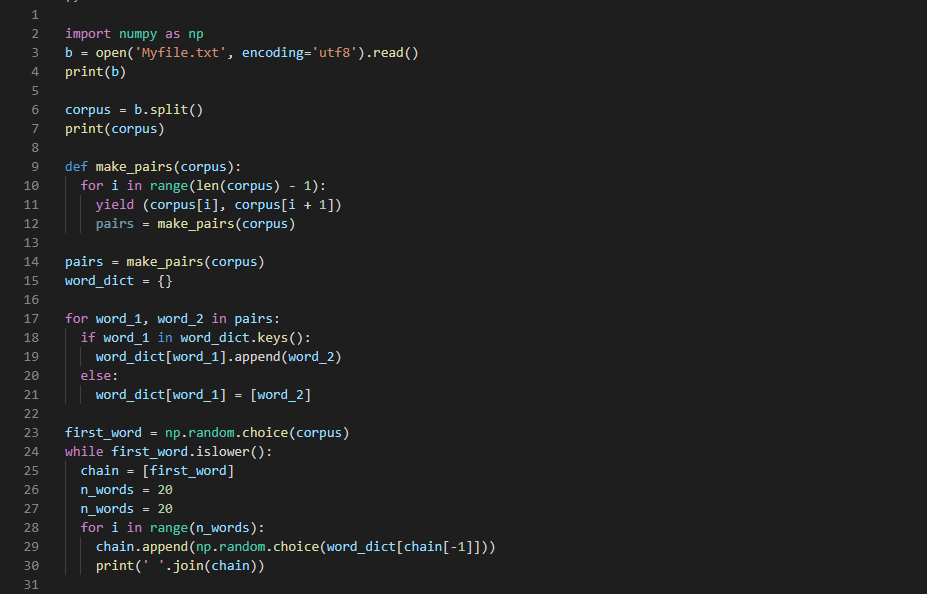### GETTING THE DATA

Before we start implementing the model, we need to get the data. I have uploaded a sample data.You can download the data on your local if you want to try on your own machine.

What Is A Transition Probability Matrix?

In the above section we discussed the working of a Markov Model with a simple example, now let’s understand the mathematical terminologies in a Markov Process.

In a Markov Process, we use a matrix to represent the transition probabilities from one state to another. This matrix is called the Transition or probability matrix. It is usually denoted by P.Transition Matrix – Introduction To Markov Chains – EzappSolution

Note, pij≥0, and ‘i’ for all values is,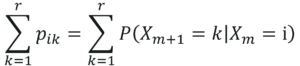Transition Matrix Formula – Introduction To Markov Chains – EzappSolution

Let me explain this. Assuming that our current state is ‘i’, the next or upcoming state has to be one of the potential states. Therefore, while taking the summation of all values of k, we must get one.

What Is A State Transition Diagram?

A Markov model is represented by a State Transition Diagram. The diagram shows the transitions among the different states in a Markov Chain. Let’s understand the transition matrix and the state transition matrix with an example.

Transition Matrix Example

Consider a Markov chain with three states 1, 2, and 3 and the following probabilities:

Transition Matrix Example - Introduction To Markov Chains - EzappSolution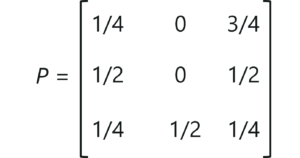State Transition Diagram Example - Introduction To Markov Chains - EzappSolution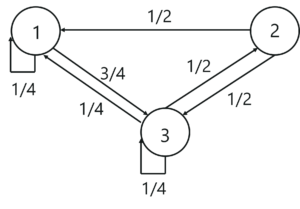The above diagram represents the state transition diagram for the Markov chain. Here, 1,2 and 3 are the three possible states, and the arrows pointing from one state to the other states represents the transition probabilities pij. When, pij=0, it means that there is no transition between state ‘i’ and state ‘j’.

Now that we know the math and the logic behind Markov chains, let’s run a simple demo and understand where Markov chains can be used.

Markov Chain In Python

Markov Chain Text Generator

Problem Statement: To apply Markov Property and create a Markov Model that can generate text simulations by studying Myfile.txt speech data set.

Data Set Description: The text file contains a list of speeches given by Myfile.txt  in 2021.

Logic: Apply Markov Property to generate Myfile’s speech by considering each word used in the speech and for each word, create a dictionary of words that are used next.

## Final Thoughts

Let’s emphasis once more how powerful Markov chains are for problems modelling when dealing with random dynamics. Due to their good properties, they are used in various fields such as queueing theory (optimizing the performance of telecommunications networks, where messages must often compete for limited resources and Markov measures are the reason for general stochastic simulation methods known as Markov chain Monte Carlo, which are utilized for simulating sampling from complex likelihood disseminations, and have found application in Bayesian statistics, thermodynamics, statistical mechanics, physics, chemistry, economics, finance, signal processing, information theory and speech processing.